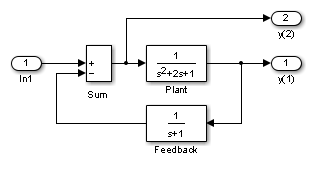Linearizing Models

The Simulink® product provides the linmod, linmod2, and dlinmod functions to extract linear models in the form of the state-space matrices A, B, C, and D. State-space matrices describe the linear input-output relationship as

$\begin{array}{l}\stackrel{˙}{x}=Ax+Bu\\ y=Cx+Du,\end{array}$

where x, u, and y are state, input, and output vectors, respectively. For example, the following model is called lmod.To extract the linear model of this system, enter this command.

[A,B,C,D] = linmod('lmod')

A =
-2    -1    -1
1     0     0
0     1    -1
B =
1
0
0
C =
0     1     0
0     0    -1
D =
0
1

Inputs and outputs must be defined using Inport and Outport blocks from the Ports & Subsystems library. Source and sink blocks do not act as inputs and outputs. Inport blocks can be used in conjunction with source blocks, using a Sum block. Once the data is in the state-space form or converted to an LTI object, you can apply functions in the Control System Toolbox™ product for further analysis:

• Conversion to an LTI object

sys = ss(A,B,C,D);

• Bode phase and magnitude frequency plot

bode(A,B,C,D) or bode(sys)

• Linearized time response

step(A,B,C,D) or step(sys)
impulse(A,B,C,D) or impulse(sys)
lsim(A,B,C,D,u,t) or lsim(sys,u,t)

You can use other functions in the Control System Toolbox and the Robust Control Toolbox™ products for linear control system design.

When the model is nonlinear, an operating point can be chosen at which to extract the linearized model. Extra arguments to linmod specify the operating point.

[A,B,C,D] = linmod('sys', x, u)

For discrete systems or mixed continuous and discrete systems, use the function dlinmod for linearization. This function has the same calling syntax as linmod except that the second right-hand argument must contain a sample time at which to perform the linearization.

Linearization with Referenced Models

You can use linmod to extract a linear model from a Simulink® environment that contains Model blocks. For example, open the referenced model mdlref_dynamics and the top model mdlref_f14.

open_system('mdlref_dynamics');
open_system('mdlref_f14');

In the mdlref_f14 model, the Aircraft Dynamics Model block references the mdlref_dynamics model.To linearize the mdlref_f14 model, use the linmod command.

[A,B,C,D] = linmod('mdlref_f14');
### Starting serial model reference simulation build
### Successfully updated the model reference simulation target for: mdlref_dynamics

Build Summary

Simulation targets built:

Model            Action                       Rebuild Reason
==========================================================================================
mdlref_dynamics  Code generated and compiled  mdlref_dynamics_msf.mexa64 does not exist.

1 of 1 models built (0 models already up to date)
Build duration: 0h 0m 30.653s

The resulting state-space model corresponds to the complete mdlref_f14 model, including the referenced model.

You can call linmod with a state and input operating point for models that contain Model blocks. When using operating points, the state vector x refers to the total state vector for the top model and any referenced models. You must enter the state vector using the structure format. To get the complete state vector, use getInitialState.

Tip

In Normal mode, the linmod command applies the block-by-block linearization algorithm on blocks inside the referenced model. If the Model block is in Accelerator mode, the linmod command uses numerical perturbation to linearize the referenced model. Due to limitations on linearizing multirate Model blocks in Accelerator mode, you should use Normal mode simulation for all models referenced by Model blocks when linearizing with referenced models.

Linearization Using the 'v5' Algorithm

Calling the linmod command with the 'v5' argument invokes the perturbation algorithm created prior to MATLAB® software version 5.3. This algorithm also allows you to specify the perturbation values used to perform the perturbation of all the states and inputs of the model.

[A,B,C,D]=linmod('sys',x,u,para,xpert,upert,'v5')
Using linmod with the 'v5' option to linearize a model that contains Derivative or Transport Delay blocks can be troublesome. Before linearizing, replace these blocks with specially designed blocks that avoid the problems. These blocks are in the Simulink Extras library in the Linearization sublibrary.

You access the Extras library by opening the Blocksets & Toolboxes icon:

• For the Derivative block, use the Switched derivative for linearization.

When using a Derivative block, you can also try to incorporate the derivative term in other blocks. For example, if you have a Derivative block in series with a Transfer Fcn block, it is better implemented (although this is not always possible) with a single Transfer Fcn block of the form

$\frac{s}{s+a}.$

In this example, the blocks on the left of this figure can be replaced by the block on the right.